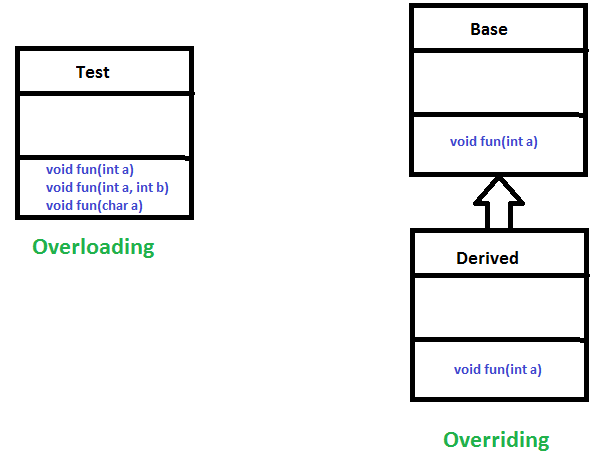# Polymorphism in Java

The word polymorphism means having many forms. In simple words, we can define polymorphism as the ability of a message to be displayed in more than one form.

Real life example of polymorphism: A person at the same time can have different characteristic. Like a man at the same time is a father, a husband, an employee. So the same person posses different behaviour in different situations. This is called polymorphism.

Polymorphism is considered as one of the important features of Object Oriented Programming. Polymorphism allows us to perform a single action in different ways. In other words, polymorphism allows you to define one interface and have multiple implementations. The word “poly” means many and “morphs” means forms, So it means many forms.

In Java polymorphism is mainly divided into two types:

• Compile time Polymorphism
• Runtime Polymorphism
1. Compile time polymorphism: It is also known as static polymorphism. This type of polymorphism is achieved by function overloading or operator overloading.• Method Overloading: When there are multiple functions with same name but different parameters then these functions are said to be overloaded. Functions can be overloaded by change in number of arguments or/and change in type of arguments.

1. Example: By using different types of arguments

 `// Java program for Method overloading ` ` `  `class` `MultiplyFun { ` ` `  `    ``// Method with 2 parameter ` `    ``static` `int` `Multiply(``int` `a, ``int` `b) ` `    ``{ ` `        ``return` `a * b; ` `    ``} ` ` `  `    ``// Method with the same name but 2 double parameter ` `    ``static` `double` `Multiply(``double` `a, ``double` `b) ` `    ``{ ` `        ``return` `a * b; ` `    ``} ` `} ` ` `  `class` `Main { ` `    ``public` `static` `void` `main(String[] args) ` `    ``{ ` ` `  `        ``System.out.println(MultiplyFun.Multiply(``2``, ``4``)); ` ` `  `        ``System.out.println(MultiplyFun.Multiply(``5.5``, ``6.3``)); ` `    ``} ` `} `

Output:

```8
34.65
```
2. Example: By using different numbers of arguments

 `// Java program for Method overloading ` ` `  `class` `MultiplyFun { ` ` `  `    ``// Method with 2 parameter ` `    ``static` `int` `Multiply(``int` `a, ``int` `b) ` `    ``{ ` `        ``return` `a * b; ` `    ``} ` ` `  `    ``// Method with the same name but 3 parameter ` `    ``static` `int` `Multiply(``int` `a, ``int` `b, ``int` `c) ` `    ``{ ` `        ``return` `a * b * c; ` `    ``} ` `} ` ` `  `class` `Main { ` `    ``public` `static` `void` `main(String[] args) ` `    ``{ ` `        ``System.out.println(MultiplyFun.Multiply(``2``, ``4``)); ` ` `  `        ``System.out.println(MultiplyFun.Multiply(``2``, ``7``, ``3``)); ` `    ``} ` `} `

Output:

```8
42
```
• Operator Overloading: Java also provide option to overload operators. For example, we can make the operator (‘+’) for string class to concatenate two strings. We know that this is the addition operator whose task is to add two operands. So a single operator ‘+’ when placed between integer operands, adds them and when placed between string operands, concatenates them.

In java, Only “+” operator can be overloaded:

• To concatenate strings

Example:

 `// Java program for Operator overloading ` ` `  `class` `OperatorOVERDDN { ` ` `  `    ``void` `operator(String str1, String str2) ` `    ``{ ` `        ``String s = str1 + str2; ` `        ``System.out.println(``"Concatinated String - "` `                           ``+ s); ` `    ``} ` ` `  `    ``void` `operator(``int` `a, ``int` `b) ` `    ``{ ` `        ``int` `c = a + b; ` `        ``System.out.println(``"Sum = "` `+ c); ` `    ``} ` `} ` ` `  `class` `Main { ` `    ``public` `static` `void` `main(String[] args) ` `    ``{ ` `        ``OperatorOVERDDN obj = ``new` `OperatorOVERDDN(); ` `        ``obj.operator(``2``, ``3``); ` `        ``obj.operator(``"joe"``, ``"now"``); ` `    ``} ` `} `

Output:

```Sum = 5
Concatinated String - joenow
```
2. Runtime polymorphism: It is also known as Dynamic Method Dispatch. It is a process in which a function call to the overridden method is resolved at Runtime. This type of polymorphism is achieved by Method Overriding.

• Method overriding, on the other hand, occurs when a derived class has a definition for one of the member functions of the base class. That base function is said to be overridden.

Example:

 `// Java program for Method overridding ` ` `  `class` `Parent { ` ` `  `    ``void` `Print() ` `    ``{ ` `        ``System.out.println(``"parent class"``); ` `    ``} ` `} ` ` `  `class` `subclass1 ``extends` `Parent { ` ` `  `    ``void` `Print() ` `    ``{ ` `        ``System.out.println(``"subclass1"``); ` `    ``} ` `} ` ` `  `class` `subclass2 ``extends` `Parent { ` ` `  `    ``void` `Print() ` `    ``{ ` `        ``System.out.println(``"subclass2"``); ` `    ``} ` `} ` ` `  `class` `TestPolymorphism3 { ` `    ``public` `static` `void` `main(String[] args) ` `    ``{ ` ` `  `        ``Parent a; ` ` `  `        ``a = ``new` `subclass1(); ` `        ``a.Print(); ` ` `  `        ``a = ``new` `subclass2(); ` `        ``a.Print(); ` `    ``} ` `} `

Output:

```subclass1
subclass2
```

Attention reader! Don’t stop learning now. Get hold of all the important DSA concepts with the DSA Self Paced Course at a student-friendly price and become industry ready.

My Personal Notes arrow_drop_upCheck out this Author's contributed articles.

If you like GeeksforGeeks and would like to contribute, you can also write an article using contribute.geeksforgeeks.org or mail your article to contribute@geeksforgeeks.org. See your article appearing on the GeeksforGeeks main page and help other Geeks.

Please Improve this article if you find anything incorrect by clicking on the "Improve Article" button below.

Improved By : bajracharyakshitij

Article Tags :
Practice Tags :

29

Please write to us at contribute@geeksforgeeks.org to report any issue with the above content.